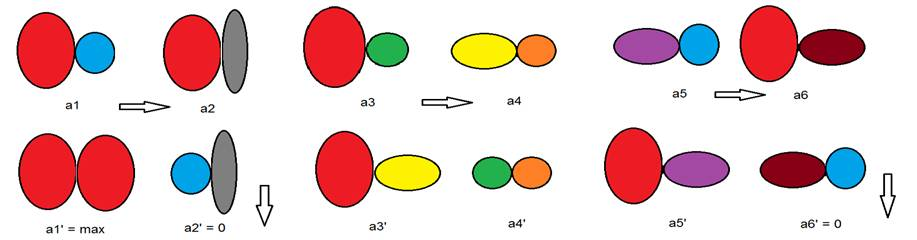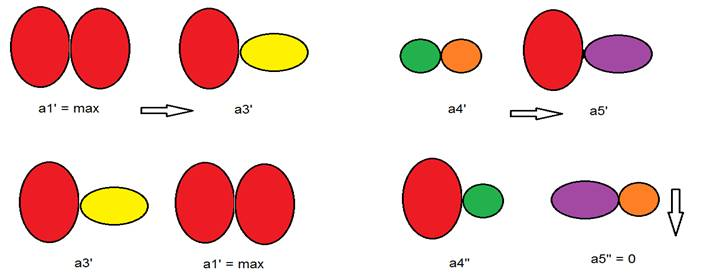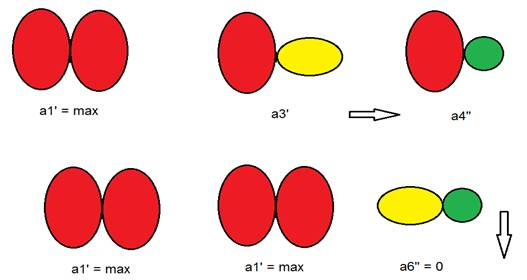5. A model hypothetical example

Let's assume, that there is population EDOCS catalyzing the same basic reaction. Suppose that at stage of 1st generation is available six variants of the duplicated structures with a miscellaneous, but low level of catalytic activity - a1, a2 .... a6. Further, suppose, that at this stage between them there was a recombination process: a1 subunits has exchanged with a2: (conditional record: a1--> a2), a3--> a4, a5--> a6.  As a result of recombination it was formed four variants with different catalytic activity, and a variant a1 ’ - with maximum activity, characteristic of symmetric structures, but two variants - with zero activity (a2' = 0, a6 '= 0).

 1-st generationVariants with zero activity die (precipitate) and the remaining EDOCS at a stage of 2nd generation re-enter the recombination: a1 '--> a3', a4 '--> a5'. As a result of this recombination another precipitating variant with zero activity (a5 "= 0) was formed.

 2-nd generationThe remaining three variants of EDOCS again come to the recombination (a stage of 3rd generations), for example a3 ”-> a4”, in which two variants of EDOCS with maximum catalytic activity (a1 '= max) and one more variant with zero activity (a6 "= 0) form. Last variant is also precipitated.

 3-d generationThe subsequent recombination has not lead to an increase in catalytic activity at EDOCS as they reach perfection (maximal symmetry and maximum power of basic reaction). However, further development can take place in more complex systems such as membranes and increase of the power of basic reaction will proceed. Already such simplified scheme shows that in the process of recombination and selection of active duplicated structures and EDOCS generational change there is a process of enrichment of population by duplicated structures with a high degree of activity and a higher symmetry. Interested persons can, by setting various parameters, to simulate this process on a computer in a much more complicated form. However, as it is possible to believe, the results of such simulations will be quite expected.

Transition to Section 6. Conclusion

Сайт создан в системе uCoz